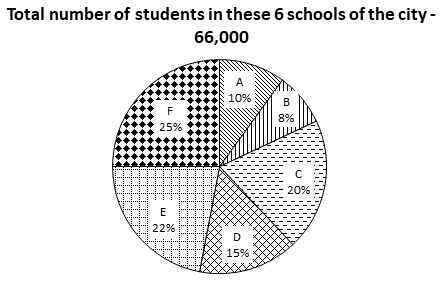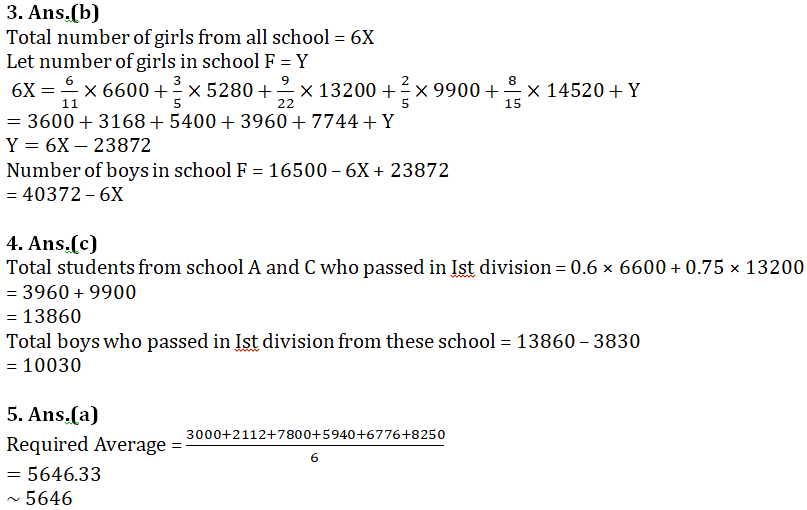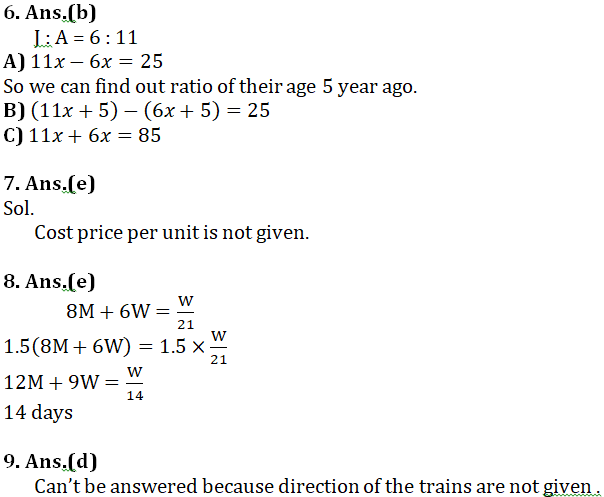# Quant Quiz (DI, DS) | 30th November 2018

Quant Quiz to improve your Quantitative Aptitude & Data Interpretation. For upcoming exams like Bank, SSC, Railway, UPSC, UPSSSC, CDS, UPTET, KVS, DSSSB and other Government exams.

Directions (1-5): Study the pie charts carefully to answer the questions that follow.

Percentage breakup of students in 6 different school of a city.Table below shows Ratio of boys students to girl students in all the school except school F. School Boy Girl A 5 6 B 2 3 C 13 9 D 3 2 E 7 8 F — —

Q1. What is the ratio of total number of boy from school A and D together to the total number of girls from school B and C together ?

(a) 853/852

(b) 223/253

(c) 745/714

(d) Can’t be determined

(e) None of these

Q2. Total number of students from school A, B and C together is approximately what percent of the total number of boys students from all school together except school F.

(a) 80%

(b) 70%

(c) 98%

(d) 110%

(e) None of these

Q3. If average of girls from all six school is ‘X’ then what is the number of boy students in school F ?

(a) 6X-23720

(b) 40372-6X

(c) 20734-6X

(d) 30249-6X

(e) None of these

Q4. If 60% of students from school A and 75% of students from school C passed in first division and total girls from both these school who passed in first division are 3830 then find the number of boys who passed in first division from these school.

(a) 9200

(b) 4800

(c) 10030

(d) 7380

(e) None of these

Q5. If number of boy and girl in school F are equal then what is the average boy students from all six schools ?

(a) 5646

(b) 4850

(c) 5152

(d) 2086

(e) 5230

Directions (6-10): The following questions are accompanied by three statements (1) , (2), and (3). You have to determine which statement(s) is/are sufficient/necessary to answer the questions.

Q6. The ratio of the ages of Javed and Akhtar is 6 :11. Find out the ratio of their ages 5 years ago.

1. The difference of their ages is 25 years.
2. The difference of their ages after 5 years will be 25 years.
3. The sum of their ages is 85 years.

(a) Only A and C together are sufficient

(b) Anyone of A, B and C is sufficient

(c) Only A and B together are sufficient

(d) Any two of A, B and C are sufficient

(e) All together are necessary

Q7. What is the cost of painting the two adjacent walls of a hall which has no windows or doors?

1. The area of the base of hall is 24 sq metres.
2. The breadth, length and the height of the hall are in the ratio of 4 : 6 : 5.
3. Area of one wall is 30 square metres.

(a) Only I

(b) Only II

(c) Only III

(d) Either I or III

Q8. 8 men and 6 women can complete a piece of work in 21 days. How many days will it take for 12 men and 9 women to complete the same work?

1. 6 men can complete the work in 42 days.
2. 7 women can complete the work in 63 days.
3. The amount of work done by a woman is four sevenths of the work done by a man in one day.

(a) Any two of them

(b) Any of them

(c) Only C

(d) Either A or B only

(e) No need of any information

Q9. A train crosses another train in 10 sec. Find out the lengths of the trains.

1. Ratio between the lengths of second and first train is 4 : 5.
2. Ratio between the speed of first and second trains is 1 : 2.
3. The speed of first train is 36 km/hr.

(a) Only A and B together

(b) Only B and C together

(c) Only A and C together

(d) Questions can’t be answered even after using all the information

(e) None of these

Q10. A customer is given two successive discounts on an article. Find the second discount.

1. The selling price of the article is given.
2. The first discount % is 30% of the second discount %.
3. The marked price of the article is given.

(a) A and B are sufficient

(b) B and C are sufficient

(c) All together are necessary

(d) All even together are not sufficient

(e) Either A and B or B and C together are sufficient

## Solutions## WhatsApp Group Join here

Mail us at : ambitiousbaba1@gmail.com